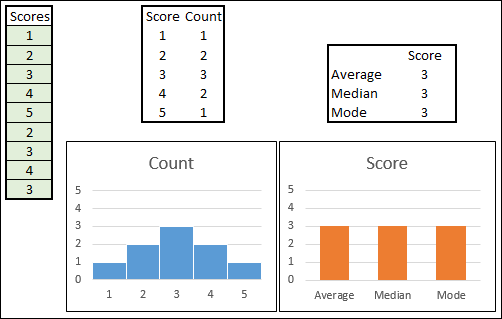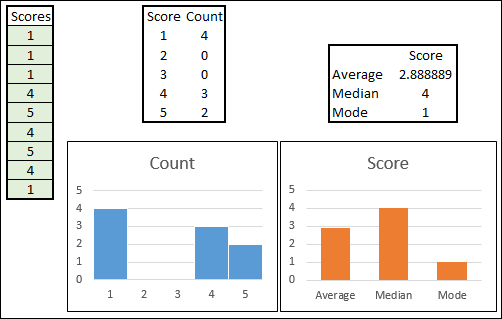# Excel Median Function Examples

Excel MEDIAN Function is one of the central tendency functions, like AVERAGE and MODE. See how these functions compare. Examples show MEDIAN function in bonus calculations and Box Plot chart setup

## Introduction

The Microsoft Excel MEDIAN function is a measure of central tendency and it returns the number in the middle of a data set.

The MEDIAN result depends on the count of numbers in the data set:

• For an odd set of numbers, the MEDIAN is the number in the middle of the sorted dataset.
• For an even set of numbers, the MEDIAN is the average of the two numbers in the middle of the sorted set.

## MEDIAN Function Example

The Excel MEDIAN function syntax has the following arguments:

MEDIAN(number1, [number2], ...)

• There is one required argument - number1
• number2 - optional argument, where another number can be entered
• Up to 255 number arguments are allowed in total

You can type numbers into the formula, or use a cell reference, or refer to a large range of cells on the spreadsheet.

#### Find Middle Number with MEDIAN

In this example, there is a small list of seven employee ID numbers, and their annual bonus payment for the current year.

Note: The list is sorted by bonus amounts, largest to smallest, but that is not necessary.

The bonus amounts are numeric values, in cells C2:C8, and the following formula is in cell E5:

• =MEDIAN(C2:C8)

There is an odd number of values in the data set, so MEDIAN returns the number in the middle of the data set - 1500.#### Find Middle Number - Even Count

In this example, there are eight employee bonus amounts in the list -- an even number of values.

The bonus amounts are in cells C2:C9, and this formula is in cell E5:

• =MEDIAN(C2:C9)

There is an even number of values in the data set, so MEDIAN returns the average of the two numbers in the middle of the data set (in cells C5 and C6) - 1350.

The AVERAGE formula in cell E2 confirms that the average of the two middle values is 1350.## MEDIAN Function - Long Lists

In the MEDIAN function arguments, there is a limit of 255 numbers allowed.

However, instead of putting individual numbers in the arguments, you can use a groups of number, in cell ranges on a worksheet. That allows you to greatly increase the count of numbers that are being evaluated.

In the screen shot below, there are two tables, and each table has a list of 1999 numbers. The total count is 3998, which is calculated in cell E2.

The MEDIAN formula in cell E5 includes both tables - ListA and ListB. The formula returns the middle number among both lists of numbers - 2000.## MEDIAN, AVERAGE and MODE

The three most commonly used measures of central tendency are Average (Mean), Median, and Mode. Here is a short comparison of the MEDIAN, AVERAGE and MODE functions in Excel.

MEDIAN Function:

• For an odd set of numbers, the MEDIAN is the number in the middle of the sorted set.
• For an even set of numbers, the MEDIAN is the average of the two numbers in the middle of the sorted set.

AVERAGE Function:

• The AVERAGE is the SUM of the numbers, divided by the COUNT of the numbers
• Learn more on the Excel Average Functions page. There are examples for AVERAGE, AVERAGEA, AVERAGEIF and AVERAGEIFS functions, as well as the TRIMMEAN function, for an average that excludes outliers.

MODE function:

• The MODE function returns the most frequently occurring number in the set
• If there aren't any duplicate numbers, the result is an #N/A error
• If there is a tie, the most frequent number that occurs first is the result

## Compare MEDIAN, AVERAGE, MODE

Below, you can see two simple examples of measuring central tendency in a small set of numbers.

There are two small sets of numbers:

Worksheet formulas calculate the AVERAGE, MEDIAN and MODE for each set, and Excel column charts show a visual summary of the results.

### Symmetrical Distribution

In the first example, the numbers are symmetrically distributed

• The green cells contain nine test scores, ranging from 1 to 5
• The Score Count list and blue column chart show the number of instances for each score
• 1 - 1
• 2 - 2
• 3 - 3
• 4 - 2
• 5 -1
• The orange column chart shows that the AVERAGE, MEDIAN and MODE are the same - 3.### Non-Symmetrical Distribution

In the next example, the numbers are NOT symmetrically distributed, as you can see in the COUNT chart.

• The green cells contain nine test scores, ranging from 1 to 5
• The Score Count list and blue column chart show the number of instances for each score
• 1 - 4
• 2 - 0
• 3 - 0
• 4 - 3
• 5 -2
• The orange column chart shows that the AVERAGE, MEDIAN and MODE are all different
• Average - 2.9
• Median - 4
• Mode - 1## Try Interactive Excel Workbook

If you would like to test the data and formulas from the above examples, try this interactive Excel workbook, shown below.

• NOTE: This might not work in all browsers.

There are two worksheets with number sets – one is symmetrically distributed, and the other is not.

To get the score counts, I used the FREQUENCY function.

## MEDIAN Example - Bonus Payment

Here is an example of using the MEDIAN function with a list of employee bonus payment amounts.

Thank you to Jonathan Cooper, who sent this example, with an unusual way to use the MEDIAN function

Jonathan had to calculate final bonus payments, based on earlier calculations (AmtA). There are 2 rules:

• The minimum bonus is zero (nobody had to give money back!)
• The maximum bonus is 1500For each employee, there are 3 possible results, 0, 1500, or AmtA. I've sorted each set of numbers, from small to large:

• Emp1: (375), 0, 1500
• Emp2: 0, 1200, 1500
• Emp3: 0, 1500, 1500
• Emp4: 0, 1500, 2775

In each case, the middle number is the amount that should be used as the final bonus calculation.

And to find the middle number in a set of numbers, you can use the MEDIAN Function.

So that's the function Jonathan used. Here's the formula in cell D8:

• =MEDIAN(B_Min,B_Max,C8)## MEDIAN Example - Box Plot Chart

To see the steps for creating a simple box plot chart, using MEDIAN and other functions, watch this short video. The written instructions are on the Excel Box Plot Chart page.

Note: To see the video transcript, go to the Box Plot Chart Video page.

For more information on the differences between the AVERAGE and MEDIAN functions, take a look at this article that compares the median and mean (average) functions.

## Get Sample File

Bonus Payment: Download the Excel workbook that shows the bonus payment calculation. The zipped file is in xlsx format, and does not contain macros.

Box Plot Chart: Download the completed Excel workbook that was used in this video -- Simple Box Plot Chart. The zipped file is in xlsx format, and does not contain macros.

## More Tutorials

Average Functions

Rounding Functions

TRIMMEAN

SUBTOTAL

Sum cells

Last updated: August 14, 2022 8:39 PM